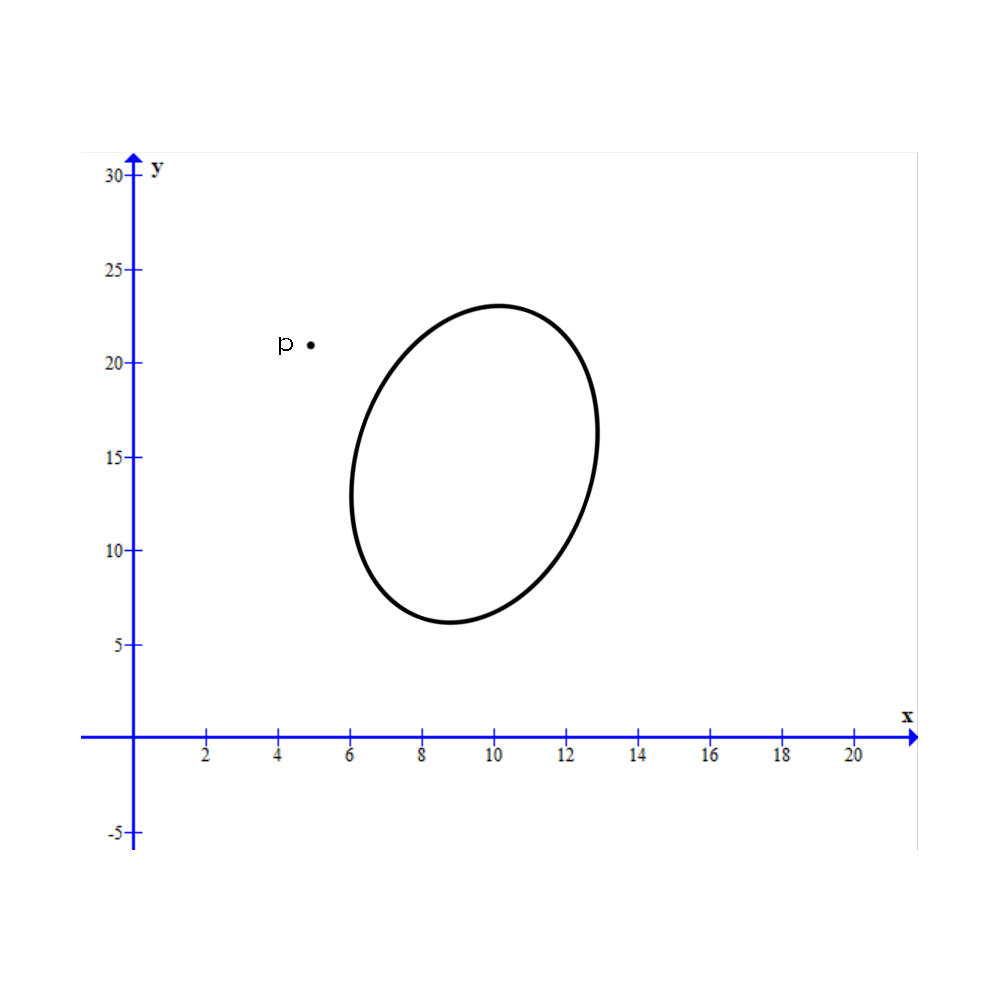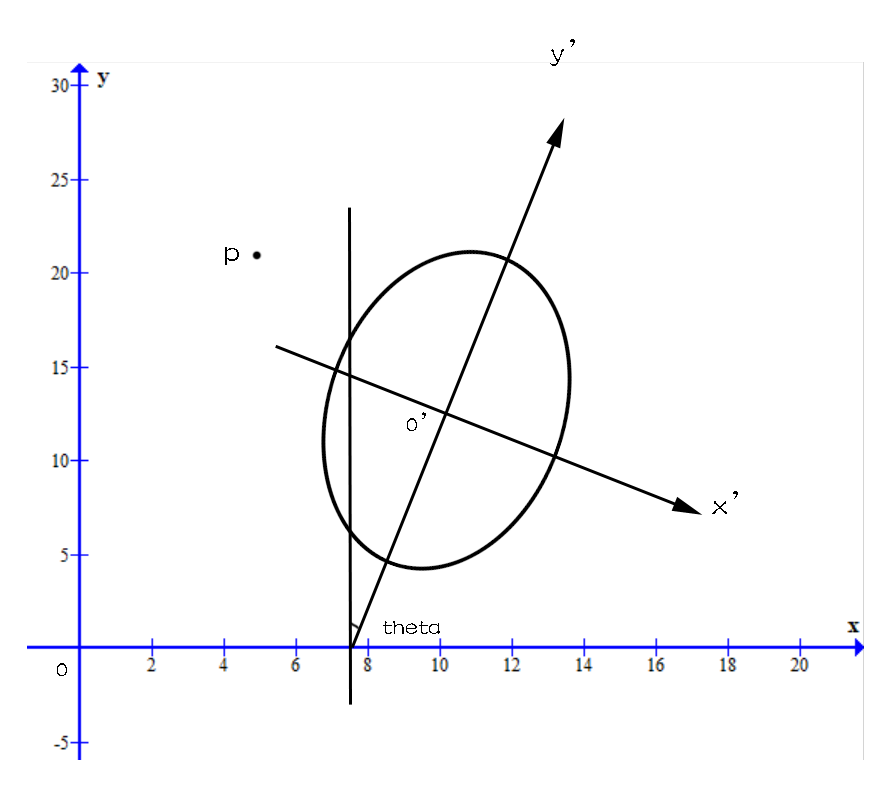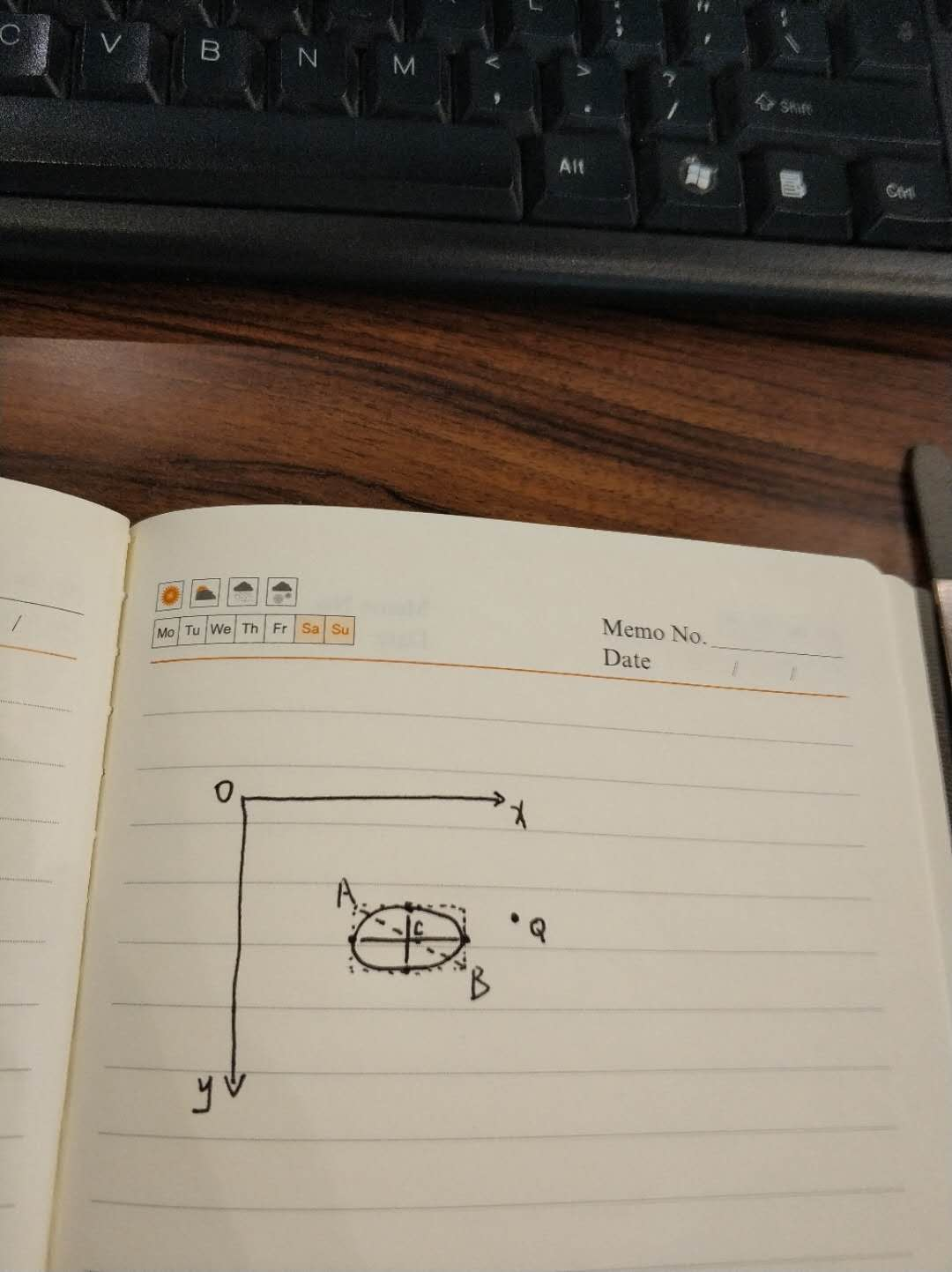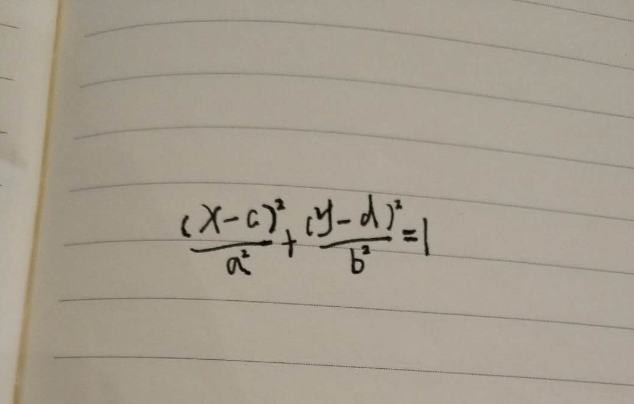• 问题描述 : 如上图所示，我们的目的是判断在二维空间中任意一椭圆与任意一点pi(xi,yi)p_i(x_i,y_i)pi​(xi​,yi​)的位置关系，这样的位置关系有三种 : 1 点在椭圆上; 2 点在椭圆中; 3 点在椭圆外。 解决思路 : 从最...
平面上任意椭圆与点的位置关系问题描述 : 如上图所示，我们的目的是判断在二维空间中任意一椭圆与任意一点$p_i(x_i,y_i)$的位置关系，这样的位置关系有三种 : 1 点在椭圆上; 2 点在椭圆中; 3 点在椭圆外。
解决思路 :
从最简单的开始讲起, 在初中时候学到过，对于一个焦点在$x$轴或$y$轴的椭圆来讲有标准方程 :
$\frac{x^2}{a^2} + \frac{y^2}{b^2} = 1$
或者
$\frac{y^2}{a^2} + \frac{x^2}{b^2} = 1$
利用标准方程，判断点与椭圆的位置关系十分容易，以焦点在$x$轴上的椭圆为例,$\forall p(x_i,y_i)\in R^2$，这里不加证明的给出位置关系的判别式 :
(1) 若
$\frac{x_i^2}{a^2} + \frac{y_i^2}{b^2} < 1$
点在椭圆内；
(2) 若
$\frac{x_i^2}{a^2} + \frac{y_i^2}{b^2} = 1$
点在椭圆上；
(3) 若
$\frac{x_i^2}{a^2} + \frac{y_i^2}{b^2} > 1$
点在椭圆外。
这一块证明的资料很多，在此就不再赘述了。

现在将问题泛化 : 对于任意的一个椭圆如何求其与点$p_i$的关系。根据上面的结论我们可以很自然的思考，如果通过一种坐标系的变换，将任意的椭圆都变为焦点在$x$轴，或$y$轴的椭圆，那么其与点$p_i$位置关系的判断将是十分容易，只需要带入已知公式即可，根据这样的思路，我们建立如下坐标系。如上图所示，在新的坐标系$x'0'y'$中，椭圆的焦点处于坐标轴上，可以使用椭圆的标准方程进行求解，唯一的问题是如何将任给一点$p_i$变换到$x'0'y'$，证明的方式有很多种，在此选用基变换.
下述方法中,变换后向量的起点$0'$的坐标是$(x_0,y_0)$点，为了满足这个条件，首先对$p_i$进行简单的平移变换，
$x_i = x_i - x_0 \\ y_i = y_i - y_0$
注 :$(x_0,y_0)$ 是椭圆圆心坐标.

求解 :
在原始坐标系$x0y$中选取一组单位正交基$\{ \vec{e_1},\vec{e_2} \},\vec{e_1} = (1,0),\vec{e_2} = (0,1)$，显然$\vec{p_i} = x_i \vec{e_1} + y_i \vec{e_2}$.
如上图先将$\vec{e_1},\vec{e_2}$旋转$\theta$角，根据向量旋转公式:
$(\vec{e_1'})^T = \begin{bmatrix} \cos(\theta)& -\sin(\theta)\\ \sin(\theta) & \cos(\theta) \end{bmatrix} \cdot \begin{bmatrix} 1 \\ 0 \end{bmatrix} \\ (\vec{e_2'})^T = \begin{bmatrix} \cos(\theta)& -\sin(\theta)\\ \sin(\theta) & \cos(\theta) \end{bmatrix} \cdot \begin{bmatrix} 0 \\ 1 \end{bmatrix}$
综上$\vec{e_1'} = \left ( \cos \theta ,\sin\theta \right ),\vec{e_2'} = (-sin\theta,cos\theta)$, 显然其满足这几点 :
(1)  $\vec{e_1'},\vec{e_2'}$ 线性无关
(2) $\vec{e_1'}\cdot\vec{e_2'} = 0$
(3) $\left | \vec{e_1'} \right | = \left | \vec{e_2'} \right | = 1$
以上通过旋转矩阵求出了新坐标系下的一组正交基，下面只需要求$\vec{p_i}$在新的基下的表示即可,解法有多种，下面展示一种通过求过度矩阵来进行求解的方法 :
将$\vec{e_1'},\vec{e_2'}$通过$\vec{e_2},\vec{e_2}$进行表示 :
$\vec{e_1'} = \cos\theta \cdot \vec{e_1} + \sin\theta \cdot \vec{e_2} \\ \vec{e_2'} = -\sin\theta \cdot \vec{e_1} + \cos\theta \cdot \vec{e_2} \\$
由上可以求出过渡矩阵
$C = \begin{bmatrix} \cos(\theta)& -\sin(\theta)\\ \sin(\theta) & \cos(\theta) \end{bmatrix}$
所以$\vec{e_1},\vec{e_2}$到$\vec{e_1'},\vec{e_2'}$的坐标变换表示为 :
$\begin{bmatrix} x_i'\\ y_i' \end{bmatrix} = C^{-1}\cdot \begin{bmatrix} x_i \\ y_i \end{bmatrix}$
整理可以得到 :
$x' = \cos\theta \cdot x + \sin\theta \cdot y \\ y' = -\sin\theta \cdot x + \cos\theta \cdot y$
上述关系式还可以通过向量间的投影关系得到.

最后将变换后的$(x'_i,y'_i)$带入判别式，计算即可。


展开全文• 在平面直角坐标系中，已知A、B两点坐标，并且画以AB为对角线的矩形的内接椭圆哦O,判断光标是否在椭圆上（选中该椭圆对象，椭圆高亮）。 首先，复习下焦点不在坐标轴的椭圆的方程： 点O(c,d)即为该椭圆中心点。 这里...
最近，在工作中遇到需要处理鼠标选中椭圆的问题，思来想去，最后老老实实使用标准方程来解决的。今天，一起假装高中生 _。
不多废话，直接上图：场景描述：
在平面直角坐标系中，已知A、B两点坐标，并且画以AB为对角线的矩形的内接椭圆哦O,判断光标是否在椭圆上（选中该椭圆对象，椭圆高亮）。
首先，复习下焦点不在坐标轴的椭圆的方程：点O(c,d)即为该椭圆中心点。
这里，我在实际项目中，为了鼠标选中椭圆明显，设置10%的上下浮动。
来看看具体实现：
bool PtInEllipse(CPoint pt1, CPoint pt2, CPoint pt0)
{
CPoint ptCenter((pt2.x + pt1.x) / 2, (pt2.y + pt1.y) / 2);

const int nLengthHorizon = abs(pt2.x - pt1.x) / 2;//a
const int nLengthVertical = abs(pt2.y - pt1.y) / 2;//b

float fRate = ((float)(pow(pt0.x - ptCenter.x, 2)) / pow(nLengthHorizon, 2)) +
((float)(pow(pt0.y - ptCenter.y, 2)) / pow(nLengthVertical, 2));

if (0.9 <= fRate&&fRate <= 1.1)//10%浮动，选中效果明显
{
return true;
}

return false;
}



展开全文• ## 标准椭圆方程推导

千次阅读 2018-01-21 18:10:49
我首先想到的办法是将被拟合点带入椭圆方程 f(x,y)=Ax2+Bxy+Cy2+Dx+Ey+Ff(x,y)=Ax2+Bxy+Cy2+Dx+Ey+Ff(x, y) =Ax^2+Bxy+Cy^2+Dx+Ey+F，如果一个点正好在椭圆上，那么 f(x,y)=0f(x,y)=0f(x, y) =0，而一个点偏离椭圆越...
初衷
用opencv拟合椭圆后，想评估一下拟合的质量，即被拟合点与拟合结果的接近程度。我首先想到的办法是将被拟合点带入椭圆方程 f(x,y)=Ax2+Bxy+Cy2+Dx+Ey+Ff(x, y) =Ax^2+Bxy+Cy^2+Dx+Ey+F，如果一个点正好在椭圆上，那么 f(x,y)=0f(x, y) =0，而一个点偏离椭圆越多，则其计算值越大，因此可以用来评估被被拟合点的偏差。 opencv 中 fitEllipse() 函数计算得到的是椭圆的中心坐标、长短轴的长度和长轴与 +x+x 轴的夹角（需要注意的是，拟合结果的 height 才是长轴），需要根据这些信息推导椭圆的标准方程。
推导
中心在原点且长轴在x轴上的椭圆方程为：x2a2+y2b2=1(1)
\dfrac{x^2}{a^2} + \dfrac{y^2}{b^2} = 1
\tag 1
其中，aa和bb分别是长半轴和短半轴。若椭圆长轴与正x轴夹角为θ\theta(逆时针)，且中心坐标为(x0,y0)(x_0,y_0)。其任意一点平移(−x0,−y0)(-x_0,-y_0)再旋转−θ-\theta即满足标准椭圆方程。
首先考虑点(x,y)(x,y)绕原点顺时针旋转角度θ\theta到(x′,y′)(x',y')。
用参数方程表示点(x,y)(x,y)和点(x′,y′)(x',y')：{x=tcos⁡ϕy=tsin⁡ϕ(2)
\begin{cases}
x = t\cos\phi\\
y = t\sin\phi
\end{cases}
\tag 2
{x′=tcos⁡(ϕ−θ)=t(cos⁡ϕcos⁡θ+sin⁡ϕsin⁡θ)y′=tsin⁡(ϕ−θ)=t(sin⁡ϕcos⁡θ−cos⁡ϕsin⁡θ)(3)
\begin{cases}
x' = t\cos(\phi-\theta)=t(\cos\phi\cos\theta+\sin\phi\sin\theta)\\
y' = t\sin(\phi-\theta)=t(\sin\phi\cos\theta-\cos\phi\sin\theta)
\end{cases}
\tag 3
因此：{x′=xcos⁡θ+ysin⁡θy′=−xsin⁡θ+ycos⁡θ(4)
\begin{cases}
x' = x\cos\theta+y\sin\theta\\
y' = -x\sin\theta+y\cos\theta
\end{cases}
\tag 4
继而可得一般椭圆方程为：[(x−x0)cos⁡θ+(y−y0)sin⁡θ]2a2+[(x−x0)sin⁡θ−(y−y0)cos⁡θ]2b2=1
\dfrac{\left[(x-x_0)\cos\theta+(y-y_0)\sin\theta\right]^2}{a^2}+
\dfrac{\left[(x-x_0)\sin\theta-(y-y_0)\cos\theta\right]^2}{b^2}=1
化简为 Ax2+Bxy+Cy2+Dx+Ey+F=0Ax^2+Bxy+Cy^2+Dx+Ey+F=0 的形式可得：A=cos⁡2θa2+sin⁡2θb2B=2sin⁡θcos⁡θ(1a2−1b2)C=sin⁡2θa2+cos⁡2θb2D=−2[cos⁡θ(x0cos⁡θ+y0sin⁡θ)a2+sin⁡θ(x0sin⁡θ−y0cos⁡θ)b2]E=−2[sin⁡θ(x0cos⁡θ+y0sin⁡θ)a2−cos⁡θ(x0sin⁡θ−y0cos⁡θ)b2]F=(x0cos⁡θ+y0sin⁡θ)2a2+(x0sin⁡θ−y0cos⁡θ)2b2−1(5)
\begin{aligned}
A=&\dfrac{\cos^2\theta}{a^2}+\dfrac{\sin^2\theta}{b^2} \\
B=&2\sin\theta\cos\theta\left(\frac{1}{a^2}-\frac{1}{b^2}\right) \\
C=&\dfrac{\sin^2\theta}{a^2}+\dfrac{\cos^2\theta}{b^2} \\
D=&-2\left[\dfrac{\cos\theta(x_0\cos\theta+y_0\sin\theta)}{a^2}+
\dfrac{\sin\theta(x_0\sin\theta-y_0\cos\theta)}{b^2}\right] \\
E=&-2\left[\dfrac{\sin\theta(x_0\cos\theta+y_0\sin\theta)}{a^2}-
\dfrac{\cos\theta(x_0\sin\theta-y_0\cos\theta)}{b^2}\right] \\
F=&\dfrac{(x_0\cos\theta+y_0\sin\theta)^2}{a^2}+
\dfrac{(x_0\sin\theta-y_0\cos\theta)^2}{b^2}-1
\end{aligned}
\tag 5

代码
在 C++ 中实现上述计算的函数为：
cv::Mat normEllipseParams(cv::RotatedRect box)
{
double params;
cv::Mat rst(6, 1, CV_64FC1, params);
double theta = box.angle / 180 * CV_PI;
double st = sin(theta);
double ct = cos(theta);
double a = box.size.width / 2;
double b = box.size.height / 2;
double a2 = a * a;
double b2 = b * b;
double x0 = box.center.x;
double y0 = box.center.y;
double xcys = x0 * ct + y0 * st;
double xsyc = x0 * st - y0 * ct;
params = ct * ct / a2 + st * st / b2;
params = 2 * st * ct * (1 / a2 - 1 / b2);
params = st * st / a2 + ct * ct / b2;
params = -2 * (ct * xcys / a2 + st * xsyc / b2);
params = -2 * (st * xcys / a2 - ct * xsyc / b2);
params = xcys * xcys / a2 + xsyc * xsyc / b2 - 1;
return rst.clone();
}

返回的 Mat 即 A~F 六个参数。
后记
测试后发现一个问题，计算椭圆方程的方法计算比较复杂，而且计算结果受椭圆大小的影响，难以直观地反应点的偏差值。后来我发现其实可以利用“椭圆上的点到其两个焦点的距离之和不变”这一性质来判断一个点偏离椭圆的程度。
椭圆的两个焦点在其长轴上，且与中心的距离为 a2+b2\sqrt{a^2+b^2};
椭圆上的点到两个焦点的距离之和为 2a2a.
计算椭圆焦点的函数为：
std::array<cv::Point2f, 2> calcFocal(cv::RotatedRect& ellipse)
{
if (ellipse.size.width < ellipse.size.height) {
swap(ellipse.size.width, ellipse.size.height);
ellipse.angle -= 90;
}
float a = ellipse.size.width / 2;
float b = ellipse.size.height / 2;
float c = sqrt(a * a - b * b);
float theta = ellipse.angle / 180 * CV_PI;
cv::Point2f delta(c * cos(theta), c * sin(theta));
return {ellipse.center + delta, ellipse.center - delta};
}

对于任意一个被拟合的点，只要计算其到两焦点的距离之和与 2a2a 的差值，即可知道它与椭圆的像素偏差大小。


展开全文• ## 椭圆相交问题

千次阅读 2013-08-16 09:04:54
我们知道，卫星通常以椭圆轨道移动，A和B也一样。但是他们的轨迹非常特殊： （1）他们的轨迹在同一平面，具有相同的圆心。 （2）连接两个焦点组成的部分互相垂直。 如果我们将中心标为O，A的焦点为F1和F2，我们就...

描述
最近天文学家发现了一对奇特的卫星，分别命名为A和B。我们知道，卫星通常以椭圆轨道移动，A和B也一样。但是他们的轨迹非常特殊： （1）他们的轨迹在同一平面，具有相同的圆心。 （2）连接两个焦点组成的部分互相垂直。 如果我们将中心标为O，A的焦点为F1和F2，我们就可以建立笛卡尔坐标，O点为圆心，通过F1和F2的为X轴。 下面是一个例子：天文学家想了解卫星更多东西，他们决定计算其相交面积。不幸的是，计算相交的面积有点难，且他们不会计算，他们求助于天才程序员的你帮忙。现在你的任务是：给定两个满足上述要求的椭圆，计算相交面积。
输入
输入包括多个测试用例。第一行为测试用例个数n（n<=100）。 在每一个测试用例包含两行，第一行A的描述轨迹，另一行描述B的轨迹，每一个描述包含两个整数a，b（a，b<=100）表示椭圆方程X2/a2+Y2/b2=1，并保证A的焦点在X轴上，B的焦点在Y轴上。
输出
对每个测试用例，用一行输出相交面积，用实型数表示，要求精确到小数点后三位。
样例输入
12 11 2
样例输出
3.709

#include<stdio.h>
#include<math.h>
const double pi=acos(-1.0);//计算圆周率
double a1,b1,a2,b2;//a1,a2和b1,b2分别是两个椭圆的x轴和y轴
double solve(){
if(a1<=a2)
return pi*a1*b1;//椭圆B包含椭圆A
if(b1>=b2)
return pi*a2*b2;//椭圆A包含椭圆B
double ans=0.0;//相交部分面积
double x,y;//两椭圆在第一象限的交点坐标
x=sqrt((b1*b1-b2*b2)*a1*a1*a2*a2/(b1*b1*a2*a2-b2*b2*a1*a1));
y=sqrt((a1*a1-a2*a2)*b1*b1*b2*b2/(a1*a1*b2*b2-b1*b1*a2*a2));
//下面计算相交部分面积
double theta1=asin(y/b1),theta2=asin(y/b2);
ans=a1*b1*((pi-2*theta1)+sin(2*theta1))+a2*b2*(2*theta2+sin(2*theta2))-4*x*y;
return ans;
}
int main(){
int n;
scanf("%d",&n);
while(n--){
scanf("%lf%lf%lf%lf",&a1,&b1,&a2,&b2);//读入数据
printf("%.3lf\n",solve());//计算相交面积
}
return 0;
}



展开全文• ## 算法系列之十三：椭圆的生成算法

万次阅读 多人点赞 2012-04-24 23:38:18
椭圆和直线、圆一样，是图形学领域中的一种常见图元，椭圆的生成算法（光栅转换算法）也是图形学软件中最常见的生成算法之一。在平面解析几何中，椭圆的方程可以描述为(x – x0)2 / a2+ (y – y0)2 / b2 = 1，其中...算法 xp
• 椭圆和直线、圆一样，是图形学领域中的一种常见图元，椭圆的生成算法（光栅转换算法）也是图形学软件中最常见的生成算法之一。在平面解析几何中，椭圆的方程可以描述为(x – x0)2 / a2+ (y – y0)2 / b2 = 1，其中...
• 首先定义了一个椭圆类用来存放一个一般化的椭圆的参数，然后构建一个椭圆方程，至于为什么需要这些参数可以参考上一篇博客：https://blog.csdn.net/qq_41685265/article/details/104267256 class Ellipse { public...opencv 计算机视觉 算法
• 引自……CSDN 已知a,b和直线上的两点，中心在原点，求直线与椭圆相交求交点坐标   #include #include #include void main() { double a,b,c,x1,x2,y1,y2,k,j; printfinput c
• 椭圆和直线、圆一样，是图形学领域中的一种常见图元，椭圆的生成算法（光栅转换算法）也是图形学软件中最常见的生成算法之一。在平面解析几何中，椭圆的方程可以描述为(x – x0)2 / a2+ (y – y0)2 / b2 = 1，其中...
• 如果一个刚体小球（碰撞不形变，无能量损失）在一个同样是刚体材料的椭圆内部无限反弹，它的轨迹会是什么样子？（也可以考虑成激光束在完美镜面椭圆内部无限反射） 爱刷抖音的朋友可能看过这样一个视频，知道答案是...算法 线性代数
•算法 加密 网络
• 第一次开始写博客，前几天学了一点jquery写了一个焦点图，一直以来，所有学的东西都是自己一个人收着，但是过了一段时间之后，再往回一看，才发现自己只不过是当初懂的而已，所以从此以后我要把自己所写的效果记录...
• 思路：若以P,Q为焦点做一椭圆椭圆与圆相交，那么交点处到P,Q的距离即为椭圆方程的2a，我们利用椭圆上任意一点到焦点的距离都为2a这一性质去找最小2a就为答案。 圆与椭圆相交的极限情况为椭圆与圆内切，此时的2a...几何
• 计算该区域内部的平均温度、最大、最小温度，圈的区域有可能是矩形、椭圆、或者任意由多条线段构成的多边形，实现这个需求可以转换为求一个点是否在该几何图形内部，下面总结一下各种几何图形的判断方法。...
• 最近做CNC插补大作业的时候，写的一段小程序，能够实现跨象限、起点（或中心）不在原点的直线、圆弧、椭圆的插补。程序已经验证无误，但是整体质量不高，作为小白(才学Python半个月左右)，希望各位大佬能够给点建议...python
• 我的2009的，我是过2011的返回的也是和你一样的不过这两种表达式应该是一样的我分别代过数值去检验，得到的结果是一样的但是我找不到办法用matlab将你得到的表达式转变成我得到的不过查了一下资料，用手推算应该可以...
• 但由于圆和椭圆有近亲关系，都是封闭曲线，且椭圆的两个焦点合二为一时，椭圆就变成了圆；双曲线和抛物线都是非封闭曲线，这两个和前两个的区别就挺大了。 基础知识 直线$$l$$和圆锥曲线$$C$$的位置关系 1、从...
• 椭圆表示一个MediaPlayer对象可以驻留在的状态。所述弧线表示驱动对象的状态过渡的重放控制操作。有两种类型的弧线。具有单箭头头部的弧线表示同步方法调用，而那些与双箭头表示异步方法调用。 MediaPlayer播放三种...
• 2005-09-01如何在VC5中控制工具条的停靠位置 - [VC专栏]作者：宋立波 在VC5 中开发大型应用程序时，工具条通常是程序中必不可少的重要功能因素， 其中工具条在整个窗口中的停靠位置尤其关键，它直接影响到程序界面的...工具 windows 框架 dropdown
• 所有不规则图形（椭圆、圆角矩形、三角形、简单的文字），最后都避不开路径绘制。 而且，若自己实现一个2D引擎，这块内容是很具有参考意义的，用OpenGL的话，图像采样等都很少关注了，对对坐标就好。但菱角、圆弧、...android skia bitmap 2d
• ## 空间统计（三）聚类分布制图

万次阅读 多人点赞 2015-03-17 15:59:43
例如，在犯罪分析中，我们可以研究哪些位置犯罪频繁并且聚集，对增设警力有重要的辅助作用。工具集中的其他工具也有类似的作用，都是通过执行聚类分析来识别具有统计显著性的热点、冷点和空间异常值的位置。依照惯例...空间统计 ArcGIS 热点分析 聚类 异常值
• 本书是讲述Delphi最经典的著作之一，作为Delphi的新版本，Delphi 6不仅能帮助程序员高效开发Windows应用程序，简化Web服务、中间软件以及后台数据库...在移动商务网络编程越来越热的今天，无疑会成为程序员关注的焦点delphi 服务器 数据库
• 1）判断点在椭圆内的公式，要写个算法，不知道用什么数据公司去判断了，在这记录下以防止自己忘了 给定椭圆f(x,y)=x^2/a^2+y^2/b^2=1, f(x1,y1)<1说明x1,y1在椭圆内部 1）焦点在X轴时，标准方程为：x^2/a^......